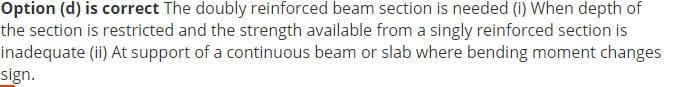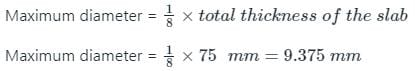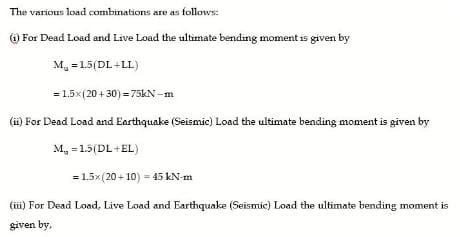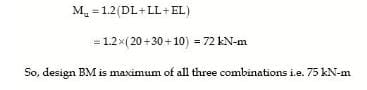# Test: Beams & Slabs - 1

## 25 Questions MCQ Test Civil Engineering SSC JE (Technical) | Test: Beams & Slabs - 1

Description
Attempt Test: Beams & Slabs - 1 | 25 questions in 50 minutes | Mock test for Civil Engineering (CE) preparation | Free important questions MCQ to study Civil Engineering SSC JE (Technical) for Civil Engineering (CE) Exam | Download free PDF with solutions
QUESTION: 1

### A doubdly reinforced beam is considered less economical than a singly reinforced beam because.

Solution:QUESTION: 2

Solution:
QUESTION: 3

### Partial safely factor for concrete and steel are 1.5 and 1.15 respectively, because

Solution:
QUESTION: 4

In the design of two-way slab restrained at all edges, torsional reinforcement required is

Solution:
QUESTION: 5

A reinforced concrete slab is 75 mm thick. The maximum size of reinforced bar that can be used is of

Solution:

As per IS 456: 2000, Clause 26.5.2.2,

Maximum Diameter:
The diameter of the reinforcing bar shall not exceed one-eighth of the total thickness of the slab.

Minimum Reinforcement:
The mild steel reinforcement in either direction in slabs shall not be less than 0.15 percent of the total cross-sectional area.

For high-strength deformed bars, the above value can be reduced to 0.12 percent.

Calculations:So, the diameter of the bars to be used should not be greater than 8 mm.

QUESTION: 6

In the limit state method, balanced design of a reinforced concrete beam gives

Solution:
QUESTION: 7

In case of simply supported beam, the clear distance between lateral restrains shall not exceed
(UP/AE 2005)

Solution:
QUESTION: 8

The prestressed concrete slab systems are ideally suited for __________

Solution:

Prestressed concrete slabs systems are ideally suited for floor and roof construction of industrial buildings where the live loads to base supported are of a higher order and the uninterrupted floor space is desirable for which reason longer span between the supporting elements are required.

QUESTION: 9

In case of 2-way slab, the limiting deflection of the slab is

Solution:
QUESTION: 10

The main reinforcement of a RC slab consists of 10 mm bars at 10 cm spacing. If it is desired to replace 10 mm bars by 12 mm bars, then the spacing of 12 mm bars should be

Solution:
QUESTION: 11

In a reinforced concrete T-beam (in which the flange is in compression). The position of neutral axis will

Solution:
QUESTION: 12

The maximum depth of neutral axis for a beam with 'd' as the effective depth, in limit state method of design for Fe 415 steel is

Solution:
QUESTION: 13

A reinforced concrete beam is subjected to the following bending moments:

The design bending moment for limit state of collapse is

Solution:QUESTION: 14

Doubly reinforced beams are recommended when

Solution:

Explanation : The depth of the beams may be restricted for architectural and/or functional requirements. Doubly reinforced beams are designed if such beams of restricted depth are required to resist moment more that its Mu.

QUESTION: 15

In a cantilever beam carrying gravity load, main reinforced is provided

Solution:
QUESTION: 16

A doubly reinforced concrete beam has effective cover 'd' to the centre of compression reinforcement. 'xu' is the depth of neutral axis, and 'd' is the effective depth to the centre of tension reinforcement. What is the maximum strain in concrete at the level of compression reinforcement?

Solution:
QUESTION: 17

Consider the following statement:

In an under-reinforced concrete beam,

1. actual depth of neutral axis is less than the critical depth of neutral axis.

2. concrete reaches ultimate stress prior to steel reaching the ultimate stress.

3. moment of resistance is less than that of balanced sections.

4. lever arm of resisting couple is less than of balanced sections.

Which of those statements is/are correct?

Solution:
QUESTION: 18

Two rectangular under-reinforced concrete beam sections X and Y are similar in all aspects except that the longitudinal compression reinforcement in section Y is 10% more. Which one of the following is the correct statement?

Solution:

Both the section i.e. x and y are under reinforced. So, in both the cases the flexural strength (MOR) can be calculated from tension side.

When additional compression reinforcement is i.e. in case of cross-section y, netural axis will shift upward.

MOR = Tension ×Lever arm

In case of cross section y lever arm is more as depth of neutral axis is less. However, tensile force is constant in both cases as the steel would have yielded in both cases. Hence flexural capacity of 'y' is more.

Also, as section y is more strong in compression, yielding in steel will be more at limit state of collapse.

Hence option (a) is corect.

QUESTION: 19

In the case of a continuous RC beam,in order to obtain the maximum positive span moment,where should the live load be placed?

Solution:
QUESTION: 20

Usually stiffness of a simply supported beam is satisfied if the ratio of its span to depth does not exceed which one of the following?

Solution:
QUESTION: 21

In a singly reinforced concrete beam section, maximum compressive stress in concrete and tensile stress reach their permissible stressess simultaneously. What is such a section called?

Solution:
QUESTION: 22

What shall be the maximum area of reinforcment

(i) in compression and

(ii) in tension to be providedin an RC beam, respectively, as per IS:456?

Solution:
QUESTION: 23

In limit state design method, the moment of resistance for a balanced section using M20 grade concrete and HYSD steel of grade Fe 415 is given by Mu,lim = Kbd2, what is the value of K?

Solution:
QUESTION: 24

How is the deflection in RC beams controlled as per IS:456?

Solution:
QUESTION: 25

What is the adoptable maximum spacing between vertical stirrups in an RCC beam of rectangular cross-section having an effective depth of 300 mm?

Solution:

Option (d) is correct As per clause 26.5.1.5 of IS : 456 – 2000, the maximum spacing of shear reinforcement measured along the axis of member shall not exceed 0.75 d for vertical stirrups and ‘d’ for inclined stirrups at 450, where ‘d’ is the effective depth of the section under  consideration. In no case shall the spacing exceed 300 mm.  Maximum spacing = 0.75 d= 0.75 x 300= 225 mmUse Code STAYHOME200 and get INR 200 additional OFF Use Coupon Code